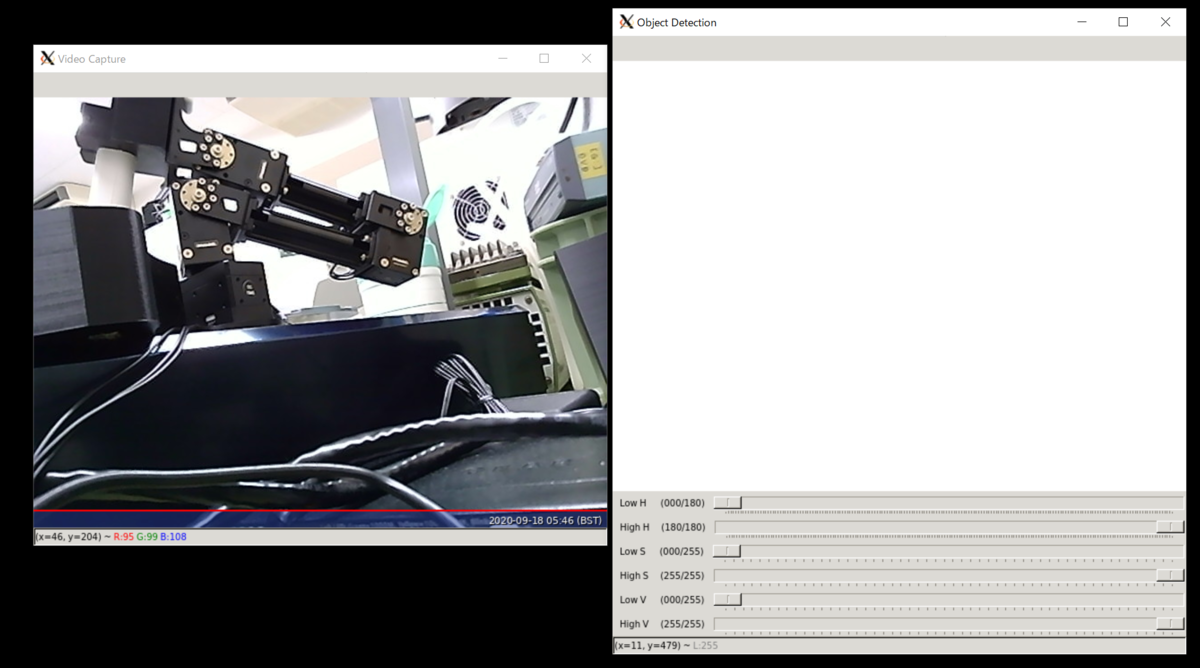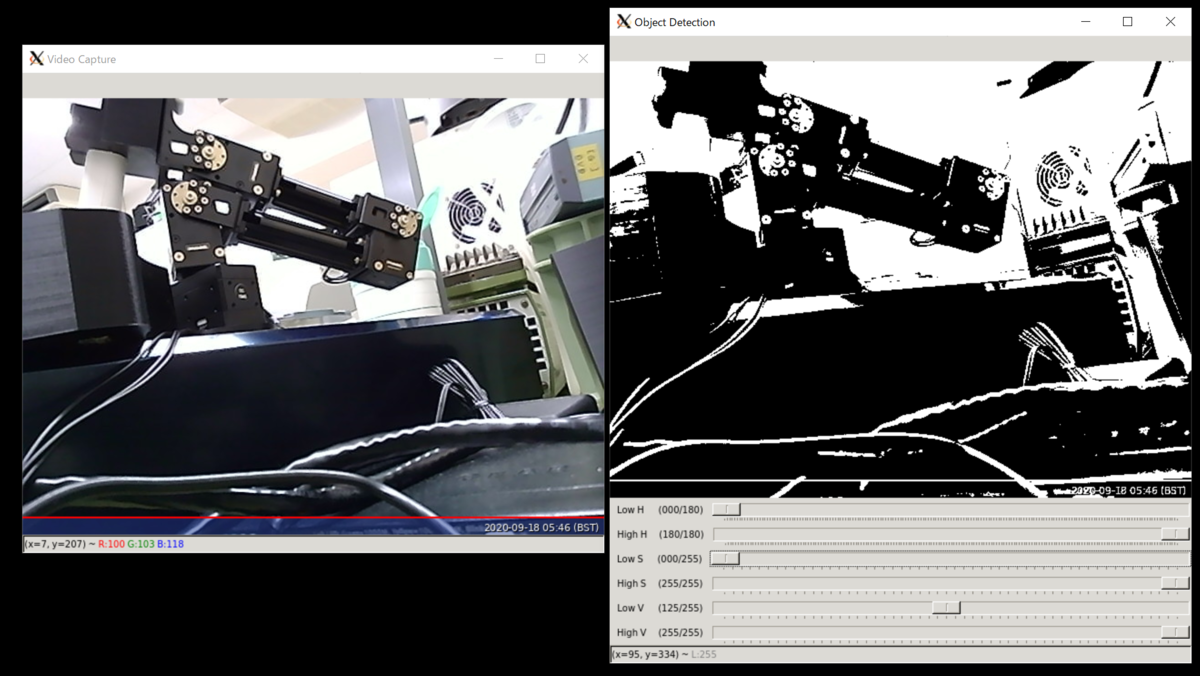# RasPi 3B+ とOpenCVによる色範囲の抽出　その１：HSV色空間のフィルタツールをやってみる

Raspberry Pi 3B+ とWaveshare社OV5648USBカメラをつかって、
MaixBit + MaixPyでやっていた事を再現するという目標。
shuzo-kino.hateblo.jp

### 実際のところ

```import cv2 as cv

max_value = 255
max_value_H = 360//2
low_H = 0
low_S = 0
low_V = 0
high_H = max_value_H
high_S = max_value
high_V = max_value
window_capture_name = 'Video Capture'
window_detection_name = 'Object Detection'
low_H_name = 'Low H'
low_S_name = 'Low S'
low_V_name = 'Low V'
high_H_name = 'High H'
high_S_name = 'High S'
high_V_name = 'High V'

def on_low_H_thresh_trackbar(val):
global low_H
global high_H
low_H = val
low_H = min(high_H-1, low_H)
cv.setTrackbarPos(low_H_name, window_detection_name, low_H)

def on_high_H_thresh_trackbar(val):
global low_H
global high_H
high_H = val
high_H = max(high_H, low_H+1)
cv.setTrackbarPos(high_H_name, window_detection_name, high_H)

def on_low_S_thresh_trackbar(val):
global low_S
global high_S
low_S = val
low_S = min(high_S-1, low_S)
cv.setTrackbarPos(low_S_name, window_detection_name, low_S)

def on_high_S_thresh_trackbar(val):
global low_S
global high_S
high_S = val
high_S = max(high_S, low_S+1)
cv.setTrackbarPos(high_S_name, window_detection_name, high_S)

def on_low_V_thresh_trackbar(val):
global low_V
global high_V
low_V = val
low_V = min(high_V-1, low_V)
cv.setTrackbarPos(low_V_name, window_detection_name, low_V)

def on_high_V_thresh_trackbar(val):
global low_V
global high_V
high_V = val
high_V = max(high_V, low_V+1)
cv.setTrackbarPos(high_V_name, window_detection_name, high_V)

cv.namedWindow(window_capture_name)
cv.namedWindow(window_detection_name)
cv.createTrackbar(low_H_name, window_detection_name , low_H, max_value_H, on_low_H_thresh_trackbar)
cv.createTrackbar(high_H_name, window_detection_name , high_H, max_value_H, on_high_H_thresh_trackbar)
cv.createTrackbar(low_S_name, window_detection_name , low_S, max_value, on_low_S_thresh_trackbar)
cv.createTrackbar(high_S_name, window_detection_name , high_S, max_value, on_high_S_thresh_trackbar)
cv.createTrackbar(low_V_name, window_detection_name , low_V, max_value, on_low_V_thresh_trackbar)
cv.createTrackbar(high_V_name, window_detection_name , high_V, max_value, on_high_V_thresh_trackbar)

while True:
frame_HSV = cv.cvtColor(frame, cv.COLOR_BGR2HSV)
frame_threshold = cv.inRange(frame_HSV, (low_H, low_S, low_V), (high_H, high_S, high_V))
cv.imshow(window_capture_name, frame)
cv.imshow(window_detection_name, frame_threshold)

key = cv.waitKey(25)
if key == ord('q') or key == 27:
cv.destroyAllWindows()
break
```スライダーを動かすと……